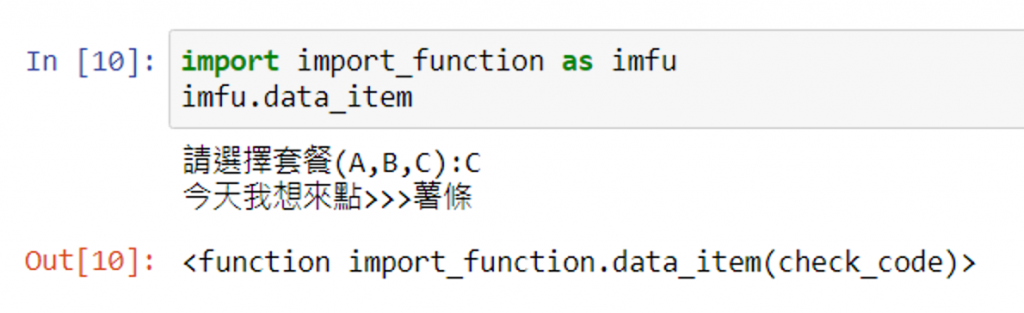#DAY 13
1
Software Development

## [2020鐵人賽Day13]糊裡糊塗Python就上手-套件模組的使用方法

### 今日目標

Python 有許多好用的套件模組，而我們今天就要來看看如何把們叫進來使用啦！

### Install package/module

Windows：
[2021鐵人賽Day04]糊裡糊塗Python就上手-Anaconda簡易操作(Windows篇)

Windows方面，安裝套件如圖示：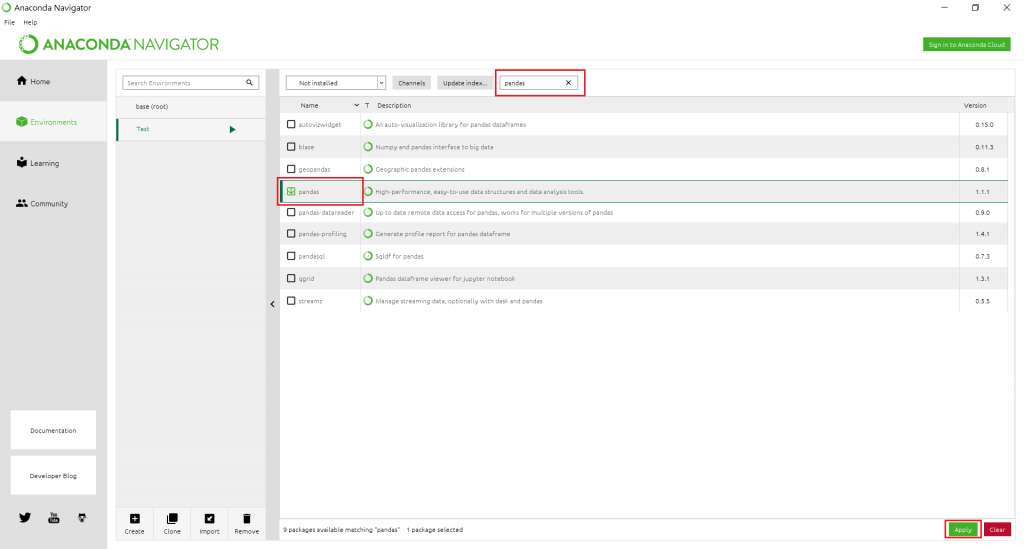Linux方面，安裝套件會有兩個方式：

``````conda install [module]
``````
``````pip install [module]
``````

Python Package Index

### Import package/module

``````import module [as identifier]
``````

``````from module import function
``````

### 實際體驗

random 模組裡的 randint 可以隨機產生出所指定範圍內的整數值

``````random.randint(a, b)
``````

``````import random as rm
print(rm.randint(50, 70))
``````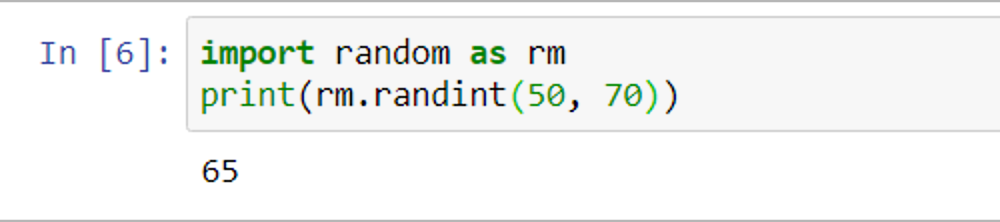``````from random import randint
print(randint(50, 70))
``````### 導入自己所寫好的 Module

``````def data_item(check_code):
if(check_code == 'A'):
return "滷肉飯"
elif(check_code == 'B'):
return "火鍋"
elif(check_code == 'C'):
return "薯條"

input_value =  input("請選擇套餐(A,B,C):")
print("今天我想來點>>>%s" %(data_item(input_value)))
``````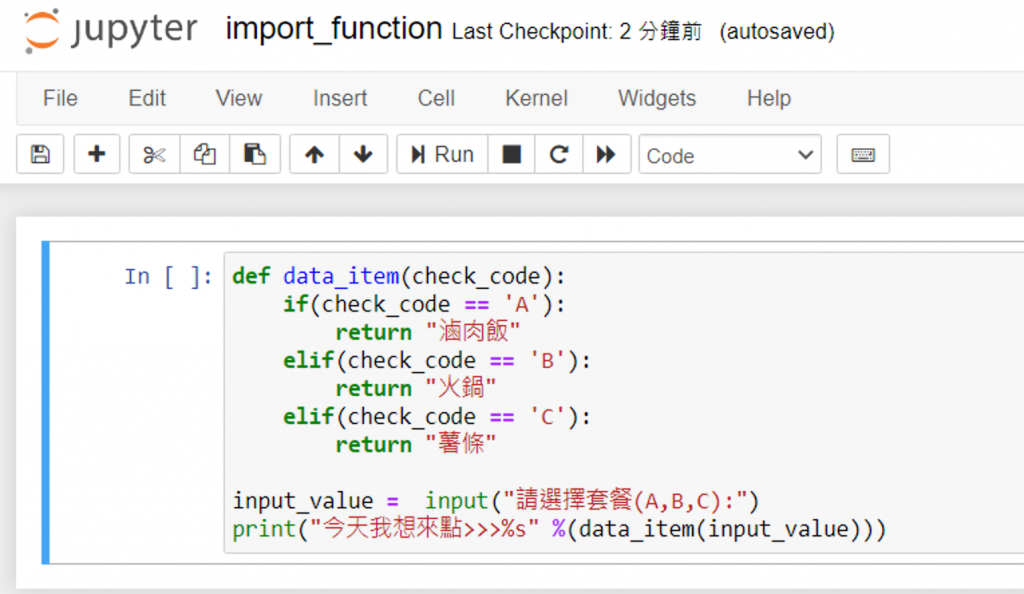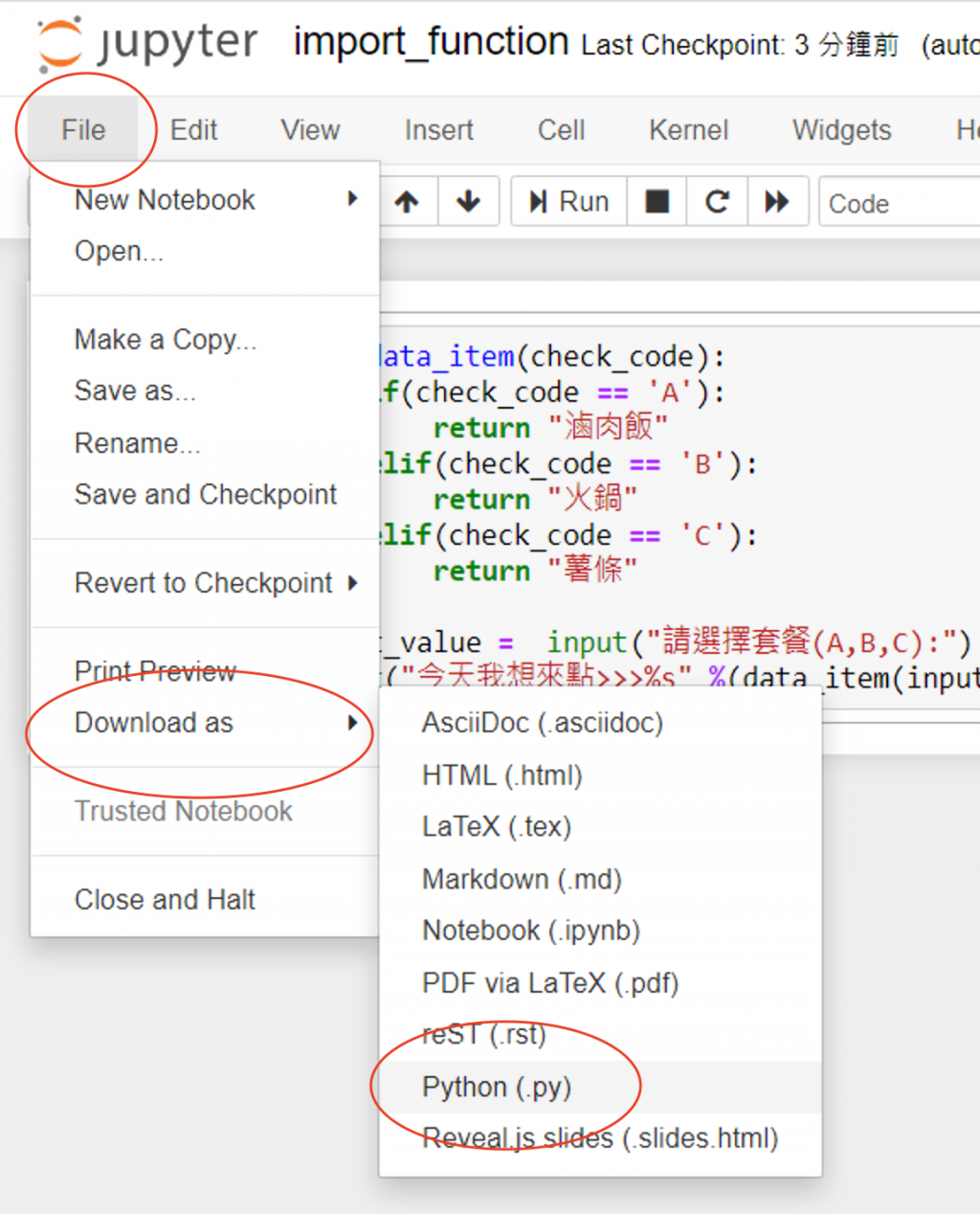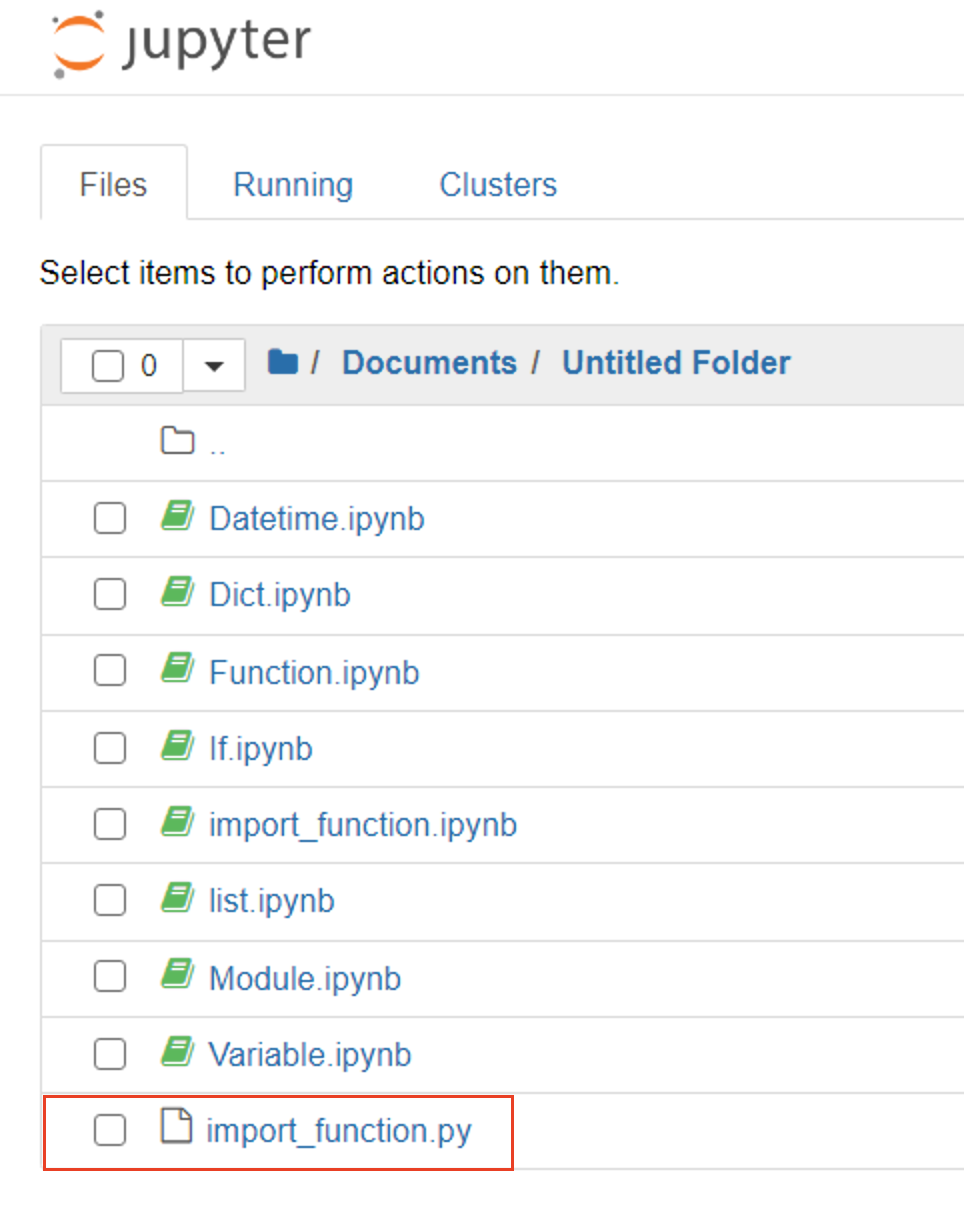``````import import_function as imfu
imfu.data_item
``````